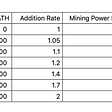# Excel Post-grouping Calculations

Introduce an easy code to deal with the difficult problems of Excel grouping

Excel supports grouping & aggregation on data, as well as offering pivot tables to perform multidimensional analysis. But Excel methods and operations are too simple to handle complicated tasks or manage them conveniently. Such tasks include getting ranks on each subset, and filtering and sorting by aggregates. In this article, we’ll give examples of post-grouping calculations, analyze it and provide SPL code solution. SPL (Structured Process Language) is the language used by esProc, the specialized data computing engine. It’s convenient to use for handling structured computations.

# I Intra-grouping sorting

Below is the student scores table. We want to get rankings for each subject. To do this we need to first group records by subject and then perform sorting on each group.

Below is the Excel data:

The expected result:

A SPL script interacts with Excel through clipboard. We edit an SPL script in esProc designer, paste the to-be-analyzed Excel data to the clipboard, execute the script, and then paste result to Excel.

SPL provides stepwise coding in an easy way:

Then we just paste the result to Excel to get the desired table.

To get records containing only the top 3 scores only for each subject, we just use top function to get the eligible records from each group.

The expected result:

SPL uses top function to get records of top 3 scores:

Then we just paste the result to Excel to get the desired table.

# II Sorting by sub-aggregate

Another scenario is to sort groups according to the sub-aggregates, such as ranking students by their total scores based on the student scores table. The expected result is as follows:

To do this, SPL uses groups function to group records while calculating total for each group:

# III Intra-group filtering

This time we want to find students whose performances are not satisfactory. Since exams of subjects are different in difficulty levels, it’s unsuitable to get them by Fail. Instead, we need to find those whose scores are below the average for each subject.

The expected result:

So we group records by subject, calculate the average of each subject, and find students whose scores are below the average.

SPL script:

# IV Filtering by sub-aggregate

In another scenario, we want to group records by name, calculate total of each group, and find students whose total scores are below the average total.

The expected result:

To perform an operation, filtering here, over the subtotals, we use groups function to group records, calculate the subtotals, get the average total and find the eligible students.

SPL script:

# V Intra-group percentage calculation

The following Excel sheet stores GDP values of some countries in 2019. The task is to group records by continent and calculate the percentage of each country’s GDP in its continent.

Below is the 2019 GDP table (Unit: \$billion):

The expected result:

We can group records by Area, sum the total GDP of each continent, and then calculate the percentage of each country’s GDP in every group.

SPL script:

# VI Calculating percentage of each group

Let’s look at how to calculate the percentage of each continent’s GDP in the global total.

The expected result:

Here we also use groups function to group records, calculate GDP of each continent, and then get the percentage of each continent’s GDP in the global total.

SPL script:

--

--

--

## More from Analytics Vidhya

Analytics Vidhya is a community of Analytics and Data Science professionals. We are building the next-gen data science ecosystem https://www.analyticsvidhya.com

## VPoS Mining Pool Tutorial 8 — The relation between MATH level and mining power## Get Started with Chef in Easy 10 Steps## Setting up SSH for multiple GIT accounts## Android CodeView the easiest way to highlight text## A Generic Connection Pool## Jerry Cruise

Products and resources that simplify hard data processing tasks. If you have any questions, send me a message. https://www.linkedin.com/in/witness998

## Introduction to Matplotlib: A library for Visualisation in Python## Using Machine Learning to Detect Skin Cancer## Concentration Guide